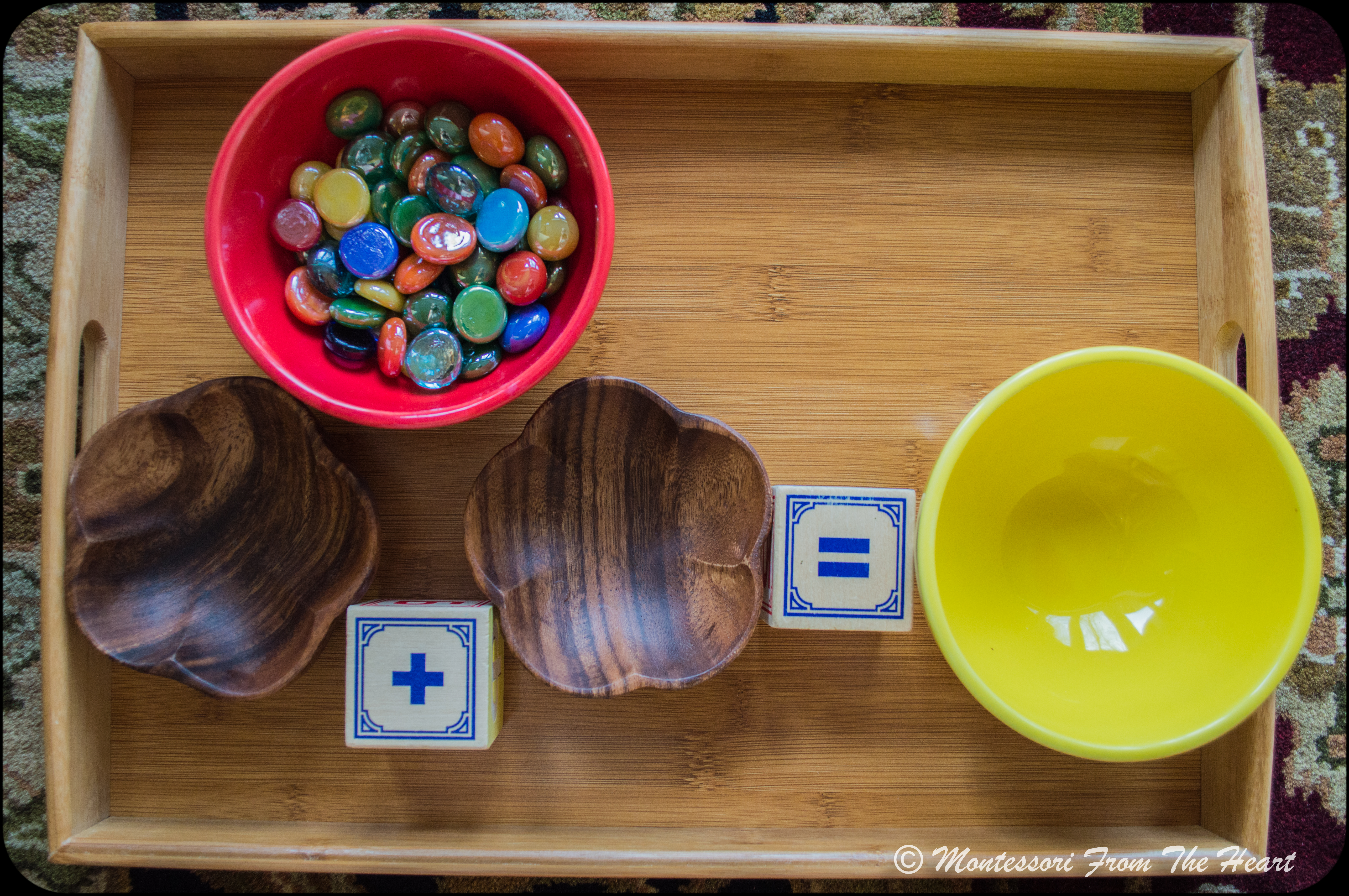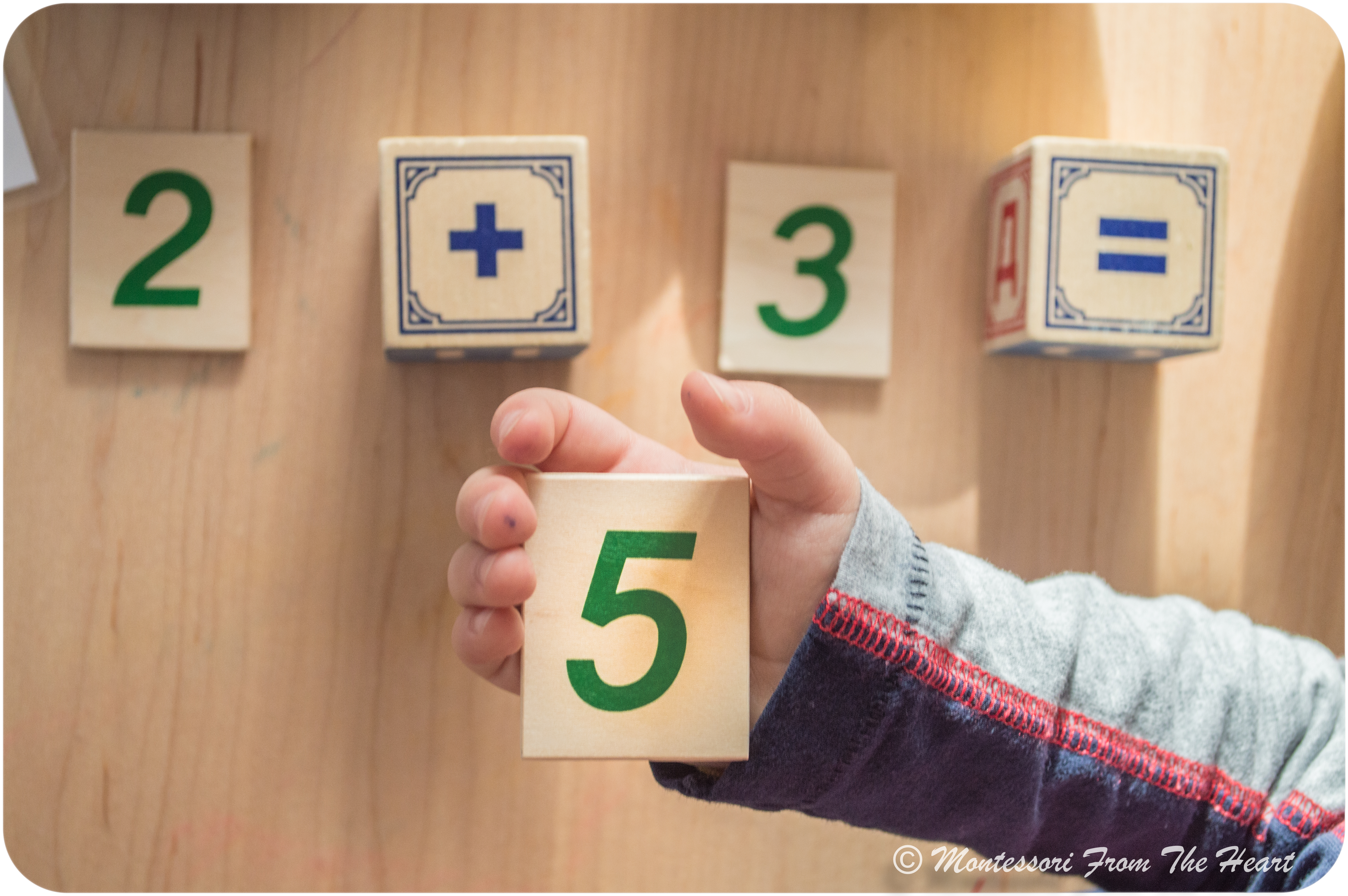What is Montessori Math?

In a traditional math curriculum, children are introduced abstract numeration before they can understand the actual quantity. Number "one"/1 is just a symbol representing a quantity (holding one apple in your hand). What is special about Montessori Math curriculum is that a three year old will understand the concept that "one" is small and "ten" is big way before any kind of numeration is introduced. Such concept is first explained in a sensorial area, where most works deal with number ten: you have ten cubes in a Pink tower, ten rods in Red Rods and Number Rods, and ten knobbed and knobless colored cylinders.

The sensorial area is where you begin to talk with your child about numbers and quantities. Maria Montessori knew that children learn by doing. Well, the math when I learned it was taught very abstractly – I simply had to trust that 3 x 5 is 15. But with Montessori math, children learn to love numbers because the understand them: for example, they understand fractions because they are building it themselves, which is a very concrete exercise.

Montessori Math lessons are build on each other just like in other areas: you would start with small concept of numbers one through 10. For example, number "one" is represented by a numeral -1 as well as by quantity when a child is holding the one apple/car/spindle in his/her hands. Such association of quantity to numeral is very concrete in nature: a child is holding one, two, or three spindles and realizes how differently it feels than holding ten. All this helps children understand in a concrete way basic mathematical concepts.This wooden transferring set (buy hereis hand-carved from native South Pacific hardwood. (Read Green Lentils Spoon Transferring Montessori Practical Life post using this set here.)

Simple addition should be presented to a child who understands the concept of numbers one through ten. This lesson uses materials you probably have at home, and it is very simple to set up.  You will need two shallow small bowls, and a different bowl for the sum. You will also need equations (I am using a chart where I wrote the equations) and counters, which can be marbles, chips, game tokens etc.

You would explain to a child that "plus" means addition – we are adding things together, and "equal" sign means the sum – what we end up with. You may download the Math Addition Template for your personal use (here) – please do not re-distribute, rather direct others to my blog.

https://player.vimeo.com/video/210521995The idea behind Montessori philosophy is to introduce the concept in the most concrete hands-on way. You would first demonstrate to the child the entire lesson. It is really important to reiterate each step (such as counting each marble even if such seems redundant) because this is how the child is learning – they watch what we do. So, you would show the lesson over and over again until a child is able to complete the entire lesson by himself.

By offering a concrete demonstration of abstract mathematical concepts, I hope to provide my child with the excitement and instill the love for learning and mathematics.

Read here a post Numeral 🔢 vs Quantity 🐮🐮 at 34 months with Self-Correcting Numbers Puzzle (Early Montessori Math).

About some of the materials mentioned in the beginning of the post: